# A concrete pedestal

A concrete pedestal has a shape of a right circular cone having a height of 2.5 feet. The diameter of the upper and lower bases are 3 feet and 5 feet, respectively. Determine the lateral surface area, total surface area, and the volume of the pedestal.

S3 =  33.836 ft2
S =  60.5395 ft2
V =  32.0704 ft3

### Step-by-step explanation: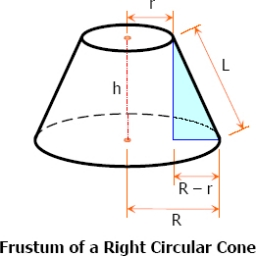Did you find an error or inaccuracy? Feel free to write us. Thank you!Tips to related online calculators
Tip: Our volume units converter will help you with the conversion of volume units.
Pythagorean theorem is the base for the right triangle calculator.

#### You need to know the following knowledge to solve this word math problem:

We encourage you to watch this tutorial video on this math problem:

## Related math problems and questions:

• Frustum of a cone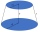A reservoir contains 28.54 m3 of water when full. The diameter of the upper base is 3.5 m, while at the lower base is 2.5 m. Find the height if the reservoir is in the form of a frustum of a right circular cone.
• Lateral surface areaThe ratio of the area of the base of the rotary cone to its lateral surface area is 3: 5. Calculate the surface and volume of the cone, if its height v = 4 cm.
• Truncated pyramidThe concrete pedestal in the shape of a regular quadrilateral truncated pyramid has a height of 12 cm, the pedestal edges have lengths of 2.4 and 1.6 dm. Calculate the surface of the base.
• Cone and the ratioThe rotational cone has a height 43 cm, and the ratio of the base surface to lateral surface is 5: 7. Calculate the surface of the base and the lateral surface.
• Surface of the coneCalculate the surface of the cone if its height is 8 cm and the volume is 301.44 cm3.
• Truncated cone 3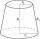The surface of the truncated rotating cone S = 7697 meters square, the substructure diameter is 56m and 42m, find the height of the tang.
• Frustrum - volume, areaCalculate the surface and volume of the truncated cone, the radius of the smaller figure is 4 cm, the height of the cone is 4 cm and the side of the truncated cone is 5 cm.
• Cone - from volume surface areaThe volume of the rotating cone is 1,018.87 dm3, and its height is 120 cm. What is the surface area of the cone?
• Truncated cone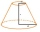Find the volume and surface area of the truncated cone if r1 = 12 cm, r2 = 5 cm and side s = 10 cm.
• Cone A2VThe surface of the cone in the plane is a circular arc with central angle of 126° and area 415 cm2. Calculate the volume of a cone.
• The diagram 2The diagram shows a cone with slant height 10.5cm. If the curved surface area of the cone is 115.5 cm2. Calculate correct to 3 significant figures: *Base Radius *Height *Volume of the cone
• The rotating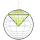The rotating cone has a height of 0.9 m and the diameter of the base is 7.2 dm. Calculate the surface of the cone. (Hint: use Pythagorean theorem for a side of cone)
• The volumeThe volume of the cone is 94.2dm³, the radius of the base is 6 dm Calculate the surface of the cone.
• Cone sideCalculate the volume and area of the cone whose height is 10 cm and the axial section of the cone has an angle of 30 degrees between height and the cone side.
• Two vases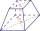Michaela has two vases in her collection. The first vase has the shape of a cone with a base diameter d = 20 cm; the second vase has the shape of a truncated cone with the lower base d1 = 25 cm and with the diameter of the upper base d2 = 15 cm. Which vas
• Truncated pyramid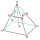The truncated regular quadrilateral pyramid has a volume of 74 cm3, a height v = 6 cm, and an area of the lower base 15 cm2 greater than the upper base's content. Calculate the area of the upper base.
• Volume of the coneCalculate the volume of the cone if the content of its base is 78.5 cm2 and the content of the shell is 219.8 cm2.